﻿ Forecasting Household Credit in Kenya Using Bayesian Vector Autoregressive (BVAR) ModelPublications are Open
Access in this journal
Article Versions
Export Article
• Normal Style
• MLA Style
• APA Style
• Chicago Style
Research Article
Open Access Peer-reviewed

Forecasting Household Credit in Kenya Using Bayesian Vector Autoregressive (BVAR) Model

Caspah Lidiema , Anthony Waititu, Thomas Mageto, Anthonyn Ngunyi
American Journal of Applied Mathematics and Statistics. 2018, 6(1), 17-24. DOI: 10.12691/ajams-6-1-4
Published online: March 28, 2018

Abstract

This research paper use Bayesian VAR framework to forecast the household credit in the dynamic market of foreign remittances inflow to Kenya. The Bayesian VARs model in this study employs the sims-Zha prior to estimate. Bayesian vector autoregressive (BVAR) uses Bayesian methods to estimate a vector autoregressive (VAR). In that respect, the difference with standard VAR models lies in the fact that the model parameters are treated as random variables, and prior probabilities are assigned to them. This study employed data from the Kenyan Market for the period January 2005-December 2017. The forecast results were compared with the standard ARIMA model and the findings confirm that the BVAR approach outperforms the ARIMA model. Financial institutions can therefore use Bayesian VAR and other Bayesian models in predicting credit uptake given several micro-economic conditions. Banks should also find ways of tapping into these remittances especially those that pass through informal channels to improve their earnings from processing fees and also enhance the financial inclusion agenda through increasing account opening and loan uptake.

1. Introduction and Literature Review

1.1. Background of the Study

Bayesian inference has grown over 300 years from its introduction by Thomas and its expansion by Richard Price, Bayes and Price 1. Bayesian inference uses prior probability distribution of an uncertain parameter say θ. This prior probability distribution expresses uncertainty about θ before taking into account the data to be used, Statisticat 2.

Vector Autoregressive (VAR) Models were proposed by Sims 3 in the paper titled “Macroeconomics and reality”. The author argued that VARs should replace large-scale macro econometric models inherited from the 1960s, because the latter imposed incredible restrictions, which were largely inconsistent with the notion that economic agents take the effect of today’s choices on tomorrows utility into account. VARs have since been used to model and forecast may macro-economic factors and often used for econometric analysis and in evaluation of proofs of economic theories, some of this studies include; Chen & Liao 4, De Medeiros et al 5 and Pecican 6.

Bayesian vector autoregressive (BVAR) is built on VAR model by employing Bayesian methods to estimate a vector autoregressive (VAR). In that respect, the difference with standard VAR models lies in the fact that the model parameters are treated as random variables, and prior probabilities are assigned to them. Sims 7 states that the objective aspect of Bayesian inference is the set of rules for transforming an initial distribution into an updated distribution condition on observations. Bayesian priors are often used to restrain the otherwise highly over-parametrized vector autoregressive (VAR) models, Villani 8.

The main advantage of Bayesian vector autoregressive (BVAR) model is that it avoids the problems of collinearity and over-parameterization that often occur with the use of VAR models since BVAR imposes priors on the AR parameters.

Remittances are funds/money sent by an expatriate of a person leaving in a foreign country to his or her home country. Due to the huge sums involved, remittances are now being recognized as an important contributor to the country’s growth and development, Central Bank of Kenya 9. However, the channels of transmission ranges from informal (unrecorded) to formal (through banks and other financial institutions). This means that that the true picture of remittances into Kenya could be much more than what is recorded by Central Bank of Kenya. While most of the remittances are believed to be channeled towards consumptions others believe that some of the remittances find its route into investment and hence boosting the economy, Meyer & Shera 10.

While foreign remittances plays a big role in reduction of poverty and enhance economic development of a country, Khan & Islam 11, credit is equally considered a key player to economic growth especially in developing nation as it lubricates the economy, Timsina 12. Equally while many researchers have agreed on the role of credit in boosting various investments options, foreign remittances has received mixed perceptions from increasing inflation, Qurbanalieva 13 to encouraging laziness. The question this paper will also try to examine is, does remittances necessitate the credit uptake borrowing from financial institutions due to increased resources to repay loans or does it hamper borrowing.

1.2. Review of Previous Studies

Mitrović & Jovičić 14 studied Macroeconomic causes and Effects of Remittances in Serbia using econometric time series, for the period December 2000 until February 2006 (63 months). All the relevant time series variables used include; remittances, output level, unemployment rate, average dollar wage, trade deficit, imports and consumer goods imports. The authors employed vector autoregressive model (VAR) with eight lags to investigate both short-run and long-run effects. The results showed that there was very strong adjustment process of consumer goods imports to the remittance inflow. They also found that appreciation of dinar is closely related to the level of remittances in the observed period and finally remittances showed an undoubtedly income increasing effect in Serbia, with their newly estimated figure reaching as much as 10% of GDP.

Meyer & Shera 10 analyzed the impact of worker remittances on economic growth of Albania and five regional countries by using annual panel data from 1999-2013. The authors used multiple regression analysis (MLR). Their results indicated that employing all necessary tests, remittances positively and significantly contribute in the economic growth of six countries. They therefore concluded that contribution of worker remittance is the significant and most important in economic growth. They further argued that the productive use of remittances can help the economy of these countries of subject to maintain and improve the economic growth by investing this money into consumption and investments.

Ramos 15 studied Forecasts of market shares using univariate Box-Jenkins, unrestricted VAR, and BVAR. The usual criteria, e.g. stationarity, autocorrelation, and partial autocorrelation functions, significance of coefficients, and the Akaike Information Criterion, were used used to select the best model. The author employed the likelihood ratio test statistic (LR) to determine the optimal lag length of the unrestricted VAR [VAR (U)]. The BVAR approach was entirely based on the likelihood function with the same Gaussian shape. The author found out that BVAR models generally produce more accurate forecasts than the ARIMA and VAR.

Caraiani 16 compared the performance of BVAR with Ordinary Least Squares LS and standard VAR in forecasting the dynamics of output for the Romanian economy. The author found out that BVAR approach outperforms the standard models (OLS and standard VAR).

Sacildi 17 investigated if BVAR Models can Forecast Turkish GDP Better Than UVAR Models. In this study the macroeconomic indicators of monetary aggregate, unemployment rate, exchange rates and interest rates are taken part in the VAR models in order to compare out-of sample forecasts of GDP by using Bayesian vector autoregressive and unrestricted vector autoregressive models. The author used quarterly data from 2005(Q4) to 2013(Q3). The BVAR models was estimated using the Minnesota/Litterman prior using different hyperparameters. The author also included the Sims and Zha prior in order to obtain more robust results. Each model’s four step ahead forecast performances are evaluated by RMSE and the results showed that BVAR model was better in accuracy for forecasting GDP than UVAR. BVAR models are also have better forecasting performance for monetary aggregate than UVAR model.

The main advantage of incorporating BVAR is because, Bayesian statistics, within a solid decision theoretical framework, incorporates a natural and principled way of combining prior information with data. Wasserman 18. This means that in our data analysis, we can incorporate past information about a parameter and form a prior distribution for current and future analysis and prediction. This usually follow from Bayes’ theorem. In fact, Robert 19 stated that “The important feature of a Bayesian approach is, thus, that Bayes estimators are derived by an eminently logical process: starting from requested properties, summarized in the loss function and the prior distribution, the Bayesian approach derives the best solution satisfying these properties”.

1.3. Statement of the Problem

Modelling Financial and economic data can be painstaking due to the dynamic nature financial markets. Quiet often financial and economic data is usually determined by previous events or previous data. As such prior information is usually carried in the current data and or future information data. Villa & Walker 20 for instance argue that Financial returns and markets index values possess extreme value characteristics and such cannot be modelled by normal distribution but models with heavier tails.

Given the fact, financial and economic data carry with it prior information, this paper This implies that, most Bayesian Vector Autoregressive Model (BVAR) is included essentially to capture prior distributions and also and improve out-of-sample performances. BVAR borrows heavily from the traditional vector Autoregressive model (VAR). The only difference is that VAR usually assume linearity and parametric nature of any financial data. The traditional VAR methods has a tendency of over-fitting and over-parameterization.

In consideration to household credit, the market dynamics of micro-economic factors could impact on credit uptake. This implies that credit uptake could be as a result of several prior market dynamics and prior borrower’s behavioral factors (credit rating, relationship with the bank and prior income). In fact, Karlsson 21 argued that “he Bayes factor captures the data evidence which can be interpreted in measuring how much our opinion about the models have changed after observing the data. The choice of model should of course take account of the losses associated with making the wrong choice”. With all this into perspective it therefore, implies the traditional methods of modelling household credit could potentially leave out information that would possibly affect the results.

This paper will therefore employ Bayesian Vector Autoregressive (BVAR) model to forecast household credit in the presence of remittances and lending rates. BVAR results will be used to compared with results of Autoregressive Integrated Moving Average (ARIMA) model which does not consider prior information.

1.4. Justification of the Study

Household credit sometimes referred to as consumer credit has gained a lot of interest in recent years. Among them is question of how household credit affects economic growth. While OECD 22 argued that Finance (credit and savings) is a key component that can spur economic growth. Maggio et al 23 believed that consumer credit contributed heavily to the 2008-2009 financial credit. This means that consumer credit expansion can negatively affect economy. Equally, remittances could affect the economy in either way (positively if the cash is invested wisely and negatively is all cash in invested in consumption hence increase money supply and hence inflation). However, the big problem is how remittances affect household credit. Brown & Carmignani 24 believe that inflow of remittances leads to changes in the credit supply and demand. This leads to financial institutions adjusting lending rates and increase profitability depending on the situation at hand. However, no empirical evidence has been carried out in Kenya to determine the true impact of remittances on household credit (That is if the receiving families, increase their loan uptake or not).This research will therefore, examine and add to the existing literature the effect of remittances on household credit while including interest rates as an intervening variable to the study. The study will be helpful to the following groups: (i) researchers and policy makers, (ii) Financial institutions, and (iii) regulators of wise monetary policies.

1.5. Objectives of the Study

The general objective of this study is to forecast household credit using Bayesian VAR model.

2. Methodology

In this study, Bayesian Vector Autoregressive Model (BVAR) will be used. The general model equation will take the form: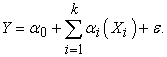(1)
2.1. Bayesian Vector Auto-regressive (BVAR) Model

From equation 1 we can re-write a general VAR (P) model equation as: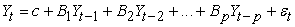(2)

where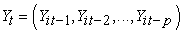is a T × N matrix i.e (Number of observations × number of endogenous variables).

In Compact form the equation above can be re-written as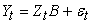where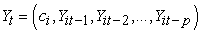is a T × (1 + N*P) matrix. ε is a white noise with zero and positive definite covariance matrix Σ or ε~iidN(0,Σ). Where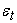is often assumed to be multivariate normal.

Bayes Law: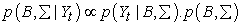(3)

where: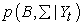is the Posterior distribution of parameters,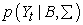is the likelihood data, and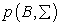is the prior distribution of parameters.

Assume that the model parameters are random variable. A prior distribution is specified based on prior information and combined with objective information from observed data to obtain the posterior distribution using Bayes Theorem. The prior distribution specification acts as a barrier, or provides 'shrinkage' preventing the estimated parameters from depicting what are only spurious correlations. One of the non-finance/non-economic studies carried out using with Bayesian VAR was by Brandt& Freeman 25 in Political science.

The likelihood function can be derived from the sampling density,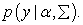As a function of the parameters, then it can be broken into two parts, that is a distribution for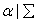and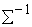which has a Wishart distribution. This are shown in equation (4) below;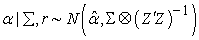(4)

Where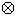represents a tensor product of two vectors.

2.1.1. The Natural Conjugate Prior

The natural conjugate prior has the form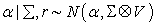(5)

and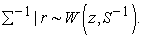(6)

The Posterior for α is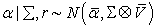(7)

where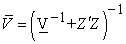and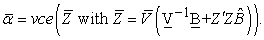The posterior for Σ is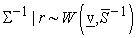(8)

where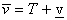and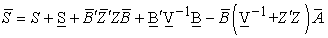2.1.2. The Independent Normal-Wishart Prior

When two or more coefficients vary, it is tedious to directly model each element of the correlation matrix. Unlike the natural conjugate prior which assumes that each equation has the same explanatory variables and restrictive prior covariance coefficients matrix in any two equations that is proportional to each other. According to Koop & Korobolis 26, the Normal-Wilshart prior is a general prior which does not involve the restrictions inherent in the natural conjugate prior.

We can therefore re-write the VAR model as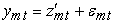(9)

where m are the variables from 1 to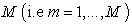and t represents the observations for the m variables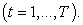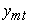is the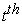observation for the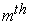variable,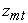is a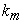vector that contains theobservation of the vector of explanatory variables relevant for thevariable and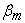is thevector of model coefficients.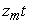is said to be unrestricted VAR if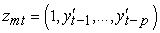for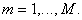However, when employing the Normal-Wilshart,can be allowed to vary across equations hence allowing the the coefficients on the lagged dependent variables to be restricted to zero (i.e allows for the possibility).

Re-writing all the equations into vectors or matrices we get;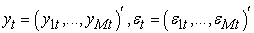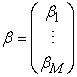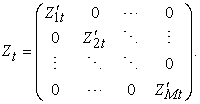As per our previous assumption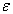is assumed to identically and independently distributed with mean Zero and Covariance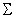(i.e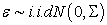).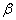is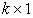matrix/vector andis an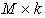Matrix and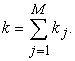From the above we can re-write our restricted VAR model as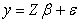(10)

where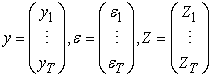and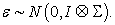This shows that the restricted VAR looks similar to Ordinary Least Squares (Linear regression model) but with with an error covariance matrix/vector.

The Independent Normal-Wilshart prior for the above model becomes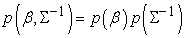(11)

where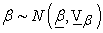(12)

and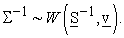(13)

We can choose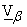to be anything for example by making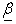andto be exact as the Minnesota prior thereby getting what is called the A noninformative prior by setting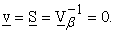From equation (13) above, we get a joint posterior of the form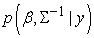which however, does not Bayesian Analysis easy. This means that the posterior mean and variance don't have any analytical form. However, Koop and Korobolis 26 stated that conditional posterior distributions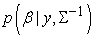and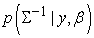do have convenient forms as shown below.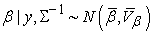(14)

To calculate valid predictions,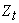should contain lags of the dependent variables, and exogenous variables which are observed at time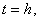where h is the desired forecast horizon. This result, along with a Gibbs sampler producing drawn for the Normal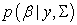and the Wilshart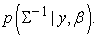Predictive simulation can be done at each Gibbs sampler draw, although this can be computationally demanding. For forecast horizons greater than one, the direct method can be used.

2.1.3. BVAR Using Sims-Zha Prior (SZBVAR)

We shall employ the estimation of the Bayesian VAR model by Sims & Zha 27. According to Adenomon et al 28, “The Sims-Zha prior allows for a more general specification and can produce a tractable multivariate normal posterior distribution”.

This BVAR model is based on a specification of the dynamic simultaneous equation representation of the model. The prior is constructed for the structural parameters. The prior covariance matrix of the errors, S(i), is initially estimated using a VAR(p) model via OLS, with an intercept and no demeaning of the data.

We can therefore re-write a m-dimensional multivariate reduced VAR model as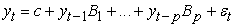(15)

where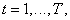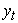is an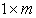matrix of observations,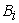is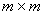matrix of coefficients for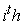lag, p is the maximum number of lags andare the residuals.

This paper employed the Independent Sims and-Zha prior distribution for the parameters in the model where the estimators are given by;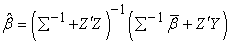(16)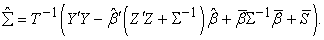(17)

Justification of prior selection: The advantage of Sims & Zha 27 approach is that it allows for a more general specification and can produce a tractable multivariate normal posterior distribution.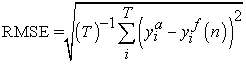(18)

where: T is the forecast of the computed values and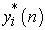is the forecasted value based on n-steps in the future.

RMSE measures the difference between values predicted by a hypothetical model and the observed values. In other words, it measures the quality of the fit between the actual data and the predicted model. For the two measures above, the smaller the value, the better the fit of the model

3. Results and Discussions

3.1. Data

The empirical data analysis is based on a set of monthly data for the period January 2005 to December 2017, Household Credit, foreign remittances into Kenya and average commercial bank lending rates. This data was obtained from Kenya Bankers Association (KBA) publication and the Central Bank of Kenya (CBK) website. The data was analyzed using R Project for Statistical computing. plotted data of Household credit and Foreign remittances is presented in Figure 1 and Figure 2 respectively. The figures depict and an exponential increasing trend in both remittances and credit.

• Figure 1. Trend of Household Credit in Kenya
• Figure 2. Trend of Remittances to Kenya

3.1.1. Results

This study employs Bayesian VAR using household credit, Remittance and Lending rates. The variables are expressed in logarithmic forms in this analysis and as such the data was not differenced to make it stationary. According to Tiriongo & Abdul 29, Sims (1980) did not recommend differencing the data, since differencing makes the data lose important information on inter-relations between variables and comovements. Several lag length options were tested and the optimal lag length of the BVAR estimation was found to be lag 2. Results of AIC for lags 1 to 10 are presented in the table below.

3.1.2. Interpretation of BVAR Results

Vector autoregressive (VAR) is a stochastic process model used to capture the linear interdependencies among multiple time series. This model framework uses the impulse response functions (IRF) and forecast error variance decomposition (FEVD) interpret the relationships between variables. IRFs are used to track the responses of a system's variables to impulses of the system's shocks. Ronayne 30. These responses are usually current and Future values for a set of variables to a one standard deviation increase in each of the variables in the system. On the other hand, forecast error variance decomposition (FEVD) is used to aid in the interpretation of a vector auto regression (VAR) model once it has been fitted. The main work for fevd is to account for variations in variables of interest overtime.

The results of the BVAR are analyzed using impulse response functions as shown in Figure 3 and Figure 4. These impulse response functions (IRFs) are created from one standard deviation shock to innovation. And the estimates are presented as per the equation (19).

• Figure 3. Response of Household Credit to Remittance
• Figure 4. Response of Household Credit to Lending rates

3.1.3. Remittance Innovations

A positive shock in remittance inflows has a significant positive effect in Household credit (Figure 3). This may reflect Increase in loan uptake. This implies that as remittances inflow increases, funds available for repayment of increase and hence borrowers are able to borrow more at will.

3.1.4. Interest rates Innovations

The innovations of lending rates decrease the loan uptake hence the negative output as shown by Figure 4. A unit basis point increase in lending rates decreases the demand for household loans. Increase in Lending rates signify a tightening monetary policy which in effect is means increase in monthly repayment and reduction money supply. Reduction in money supply in the market means hinders loan uptake due to difficulty to meat repayment schedules.

The model equation is then presented as follows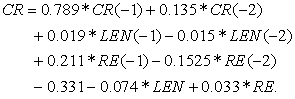(19)
3.2. Model Estimation Comparison

This research study employs Akaike Information Criteria (AIC) to select the best model in estimation. AIC is chosen because it is easier to interpret. AIC is generally used to estimate the information loss and the best model is the one the loses the lowest information. AIC is generally given as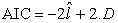(20)

where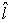is the maximum likelihood for the model, and D is the the free parameter (otherwise known as degrees of freedom).

The author also compared the AIC and BIC with a view to find out if the results would be different. Dziak et al 31 proposed the use of both AIC and BIC in model selection since AIC could perform better in scenarios where a false negative is considered more misleading than a false positive. BIC on the other hand is would preferred better where a false positive is is more misleading than a false negative.

BIC is denoted as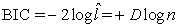(21)

whereis the maximum likelihood for the model, and D is the the free parameter (otherwise known as degrees of freedom). The main advantage of BIC is its consistent as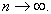From estimation results both AIC and BIC performed the same in model selection as indicated in Table 2, while ARIMA performed better than BVAR in estimation, BVAR outperformed ARIMA in prediction and forecasting as shown in Table 2.

3.3. Predictive Ability

The last objective of this research is to compare the models in terms of prediction performance in order to make a decision on the forecasting accuracy of the models. The forecasting performance is evaluated root mean square errors (RMSE).

The RMSE is given by: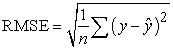(22)

Where y is the actual value and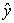is the forecasted one. This is essentially the sample standard deviation of the forecast errors (without any degrees of freedom adjustment).

The results indicate that the ARIMA model outperforms the BVAR estimation using AIC and BIC results. However, BVAR outperforms ARIMA model in predictive accuracy as indicated in Table 2 above. Wooldridge 32 confirms that a model might provide a good fit to a response variable in the sample used to estimate the parameters, but this need not translate to good forecasting performance. This results also confirms that Bayesian Vector Autoregressive models are good in predictive ability due to the nature of using priors in the model. Bayesian Models are also multivariate in nature unlike ARIMA model which is univariate in nature.

4. Conclusion and Recommendations

The exponential increase in foreign remittances has led to speculation in academia and the financial sectors research to examine the extent to which theses remittances affect the economy. While many studies have centered on the economy (GDP) little research has been carried out on how remittances affect household credit. This research was therefore geared to determine the extent to which Bayesian VAR performs in forecasting household credit in relation to foreign remittances. It's difficult to forecast credit unilaterally due to the dynamic nature of credit uptake affected by liquidity, inflation, lending rates and above all behavioral factors of borrowers, and hence the reason for this paper to introduce foreign remittances in the model. This paper contributes to the growth of using Bayesian models which has seen greater expansion in finance in recent times to forecast household credit in Kenya. The Bayesian VAR used in this paper was compared with ARIMA model on forecasting accuracy. The results indicate that the BVAR models outperforms the ARIMA model in predicting household credit given foreign remittances.

The results could also be an indicator of how remittances impact of financial inclusion agenda in the country. If increased remittances leads to increased household credit, then it implies that at least from the formal channels, people are expected to not only open new accounts but take credit also and hence contribute to financial inclusion agenda.

Banks, investors and other financial market players can therefore employ Bayesian models in predicting credit uptake given several micro-economic factors including foreign remittances. Given the effects of foreign remittances on household credit, financial institutions should also use this study to enhance tapping foreign remittances from informal channels so as to increase their revenue through processing fees and also improve their short term liquidity. Researchers should expand this study by incorporating other factors not captured in this study and compare the results. A separate study should also be carried out to determine to what extent does remittances contribute to the Financial inclusion in Kenya.

References

  Bayes T, Price R (1763). “An Essay Towards Solving a Problem in the Doctrine of Chances. By the late Rev. Mr. Bayes, communicated by Mr. Price, in a letter to John Canton, MA. and F.R.S.” Philosophical Transactions of the Royal Society of London, 53, 370-418. In article View Article  Statisticat, L. L. C. (2016). Laplaces Demon: Complete Environment for Bayesian Inference. R package version 16.0. 1. In article  Sims CA. 1980. Macroeconomics and reality. Econometrica 48: 1-48. In article View Article  Chen, F. Y., & Liao, S. L. (2009). Modelling VaR for foreign-asset portfolios in continuous time. Economic Modelling, 26(1), 234-240. In article View Article  De Medeiros, O. R., Van Doornik, B. F. N., & de Oliveira, G. R. (2011). Modeling and forecasting a firm's financial statements with a VAR-VECM model. CEP, 70843, 030. In article View Article  Pecican, E. S. (2010). Forecasting based on open VAR model. Romanian Journal of Economic Forecasting, 13(1), 59-69. In article View Article  Sims (2007) Bayesian Methods in Applied Econometrics, or, Why Econometrics Should Always and Everywhere Be Bayesian. Technical Report, Princeton University. In article View Article  Villani, M. (2009). Steady-state priors for vector autoregressions. Journal of Applied Econometrics, 24(4), 630-650. In article View Article  https://www.centralbank.go.ke/diaspora-remittances/. In article View Article  Meyer, D., & Shera, A. (2017). The impact of remittances on economic growth: An econometric model. Economi A, 18(2), 147-155. In article View Article  Khan, Z. S., & Islam, S. (2013). The effects of remittances on ination: evidence from Bangladesh. Journal of Economics and Business Research, 19(2), 198-208. In article  Timsina, N. (2014). Impact of bank credit on economic growth in Nepal. NBER Working Paper, (22). In article View Article  Qurbanalieva, N. (2013). An empirical study of factors affecting ination in Republic of Tajikistan. Journal of Applied Economic Sciences Quarterly, ASERS Publishing, 2, 229-246. In article  Dragutinovic Mitrovic, R., & Jovičić, M. (2006). Macroeconomic analysis of causes and effects of remittances: a panel model of the SEE countries and a case study of Serbia (No. 63). The Vienna Institute for International Economic Studies, wiiw. In article  Ramos, F. F. R. (2003). Forecasts of market shares from VAR and BVAR models: a comparison of their accuracy. International Journal of Forecasting, 19(1), 95-110. In article View Article  Caraiani, P. (2010). Forecasting Romanian GDP using BVAR model. Romanian Journal of Economic Forecasting,13(4),76-87 In article View Article  Sacakli-Sacildi, I. (2015), Do BVAR Models Forecast Turkish GDP Better Than UVAR Models? British Journal of Economics, Management & Trade. 7(4), 259-268. In article View Article  Wasserman, L. (2004). All of Statistics: A Concise Course in Statistical Inference. Springer Science & Business Media. In article View Article  Robert, C. P. (2001), The Bayesian Choice, Second Edition, New York: Springer-Verlag. In article  Villa, C., & Walker, S. G. (2014). Objective prior for the number of degrees of freedom of at distribution. Bayesian Analysis, 9(1), 197-220. In article View Article  Karlsson, Sune (2015). Forecasting with Bayesian Vector Autoregression. Handbook of Economic Forecasting. 2 B: 791-897 In article View Article  OECD (2015), “How to restore a healthy financial sector that supports long-lasting, inclusive growth?”, OECD Economics Department Policy Notes, No. 27, June 2015. In article  Di Maggio, M., Kermani, A., Ramcharan, R., & Yu, E. G. (2017). Household Credit and Local Economic Uncertainty. https://papers.ssrn.com/sol3/papers.cfm?abstract_id=2991227. In article View Article  Brown, R. P., & Carmignani, F. (2015). Revisiting the effects of remittances on bank credit: a macro perspective. Scottish Journal of Political Economy, 62(5), 454-485. In article View Article  Brandt, P. T., & Freeman, J. R. (2005). Advances in Bayesian time series modeling and the study of politics: Theory testing, forecasting, and policy analysis. Political Analysis, 14(1), 1-36. In article View Article  Koop, G., & Korobilis, D. (2010). Bayesian multivariate time series methods for empirical macroeconomics. Foundations and Trends in Econometrics, 3(4), 267-358. In article View Article  Sims, Christopher A. and Tao A. Zha. 1998. “Bayesian Methods for Dynamic Multivariate Models.” International Economic Review 39(4): 949-968. In article View Article  Adenomon, M. O., Michael, V. A., & Evans, O. P. (2016). On the Performances of Classical VAR and Sims-Zha Bayesian VAR Models in the Presence of Collinearity and Autocorrelated Error Terms. Open Journal of Statistics, 6(01), 96-132. In article View Article  Samuel, T., & Abdul, F. (2017). Policy Innovations and Sectoral Credit Expansion in Kenya. KBA Working paper Series, 20. In article View Article  Ronayne, D. (2011). Which impulse response function?. In article View Article  Dziak, JJ., Cofiman, D.L., Lanza, S.T., & Li, R. (2017). Sensitivity and Specificity of information criteria. Peer J Pre Prints. In article  Wooldridge, J. M. (2015). Introductory econometrics: A modern approach. Fourth Edition. Nelson Education. In article

Published with license by Science and Education Publishing, Copyright © 2018 Caspah Lidiema, Anthony Waititu, Thomas Mageto and Anthonyn NgunyiThis work is licensed under a Creative Commons Attribution 4.0 International License. To view a copy of this license, visit http://creativecommons.org/licenses/by/4.0/